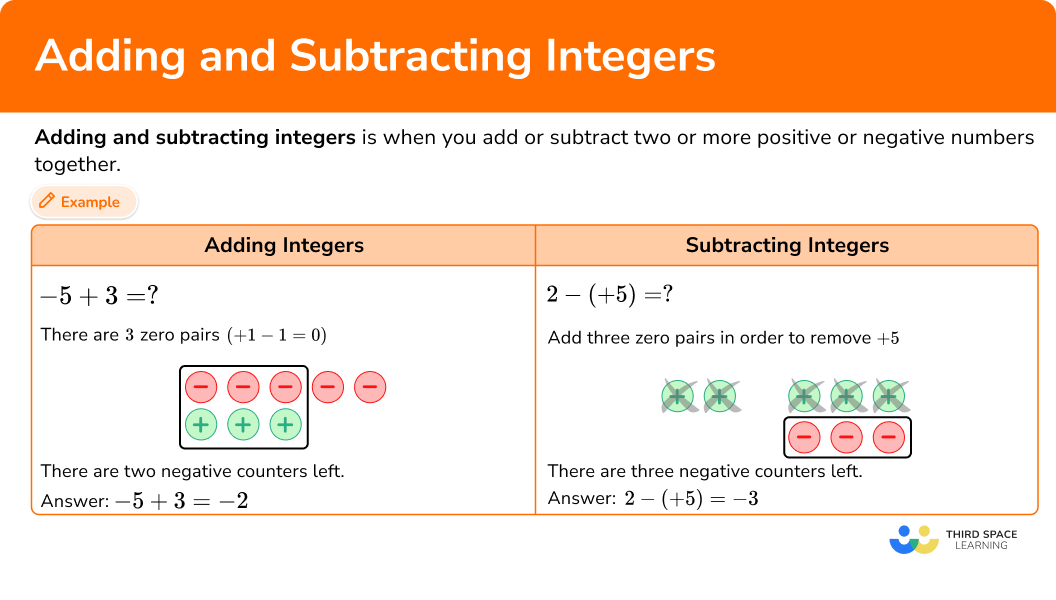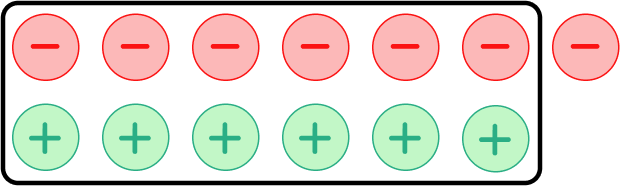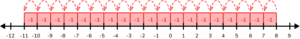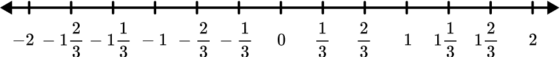Here you will learn strategies on how to add and subtract integers, including using visual models as well as the number line.

Students will first learn about integers in 6th grade math as part of their work with the number system and expand that knowledge to operations with integers in the 7th grade.

## What are adding and subtracting integers?

Adding and subtracting integers is when you add or subtract two or more positive or negative numbers together.

You can add and subtract integers using visual models or a number line.

Visual model with counters:
3 + 2 = \, ?

There are no zero-pairs.
There are 5 positive pieces.

Number line:
3 + 2 = \, ?

Start at 3 and move 2 places in the
positive direction. You land at 5.

Visual model with counters:
-5 + 3 = \, ?

There are 3 zero pairs

There are two negative counters left.

Answer: -5 + 3 = -2

Number line:
-5 + 3 = \, ?

Start at -5 and move three places in
the positive direction. You land at -2.

Answer: -5 + 3 = -2

Visual model with counters:
6 + (-2) = \, ?

There are two zero pairs with four
positive counters left.

Answer: 6 + (-2) = 4

Number line:
6 + (-2) = \, ?

Start at 6 and move two places in
the negative directions. You land at 4.

Answer: 6 + (-2) = 4

Visual model with counters:
-3 + (-4) = \, ?

There are no zero pairs. There are
7 negative counters all together.

Answer: -3 + (-4) = -7

Number line:
-3 + (-4)= \, ?

Start at -3 and move 4 places in the
negative direction. You land on -7.

Answer: -3 + (-4) = -7

Subtracting Integers \hspace{2cm}

Visual model with counters:
-3 - (-2) = \, ?
-3 take away -2

There are three negative counters.
Taking away two of them, leaves you
with 1 negative counter.

Number line:
-3-(-2) means from -2 to -3 on
the number line.

From -2 move left one unit to -3,
which is -1 unit.

Visual model with counters:
-3-(+2) = \, ?
-3 take away +2. Add two zero pairs
in order to remove +2.

There are five negative counters left.

Number line:
-3-(+2) means from +2 to -3 on
the number line.

From +2 move left 5 units to -3,
which is -5 units.

Visual model with counters:
2-(+5) = \, ?

2 take away +5. Add three zero pairs
in order to remove +5.

There are three negative counters left.

Number line:
2-(+5) = ?

2-(+5) means from +5 to +2 on
the number line.

From +5 move left three units to +2,
which is -3 units.

### What are adding and subtracting integers?## Common Core State Standards

How does this apply to 6th grade math and 7th grade math?

• Grade 6: Number System (6.NS.C.6)
Understand a rational number as a point on the number line. Extend number line diagrams and coordinate axes familiar from previous grades to represent points on the line and in the plane with negative number coordinates.

• Grade 7: Number System (7.NS.A.1)
Apply and extend previous understandings of addition and subtraction to add and subtract rational numbers; represent addition and subtraction on a horizontal or vertical number line diagram.

## How to add and subtract integers?

In order to add and subtract integers using counters:

1. Represent the problem with counters identifying zero pairs with addition or adding zero pairs when necessary for subtraction.
2. The answer is the leftover counters.

In order to add and subtract integers using a number line:

1. To add, start at the first number and move to the second number; to subtract, start from the second number and move to the first number.

## Adding and subtracting integers examples

### Example 1: adding integers with different signs using counters

Add: -2 + 7 = \, ?

1. Represent the problem with counters, identifying zero pairs with addition or adding zero pairs when necessary for subtraction.

There are two zero pairs with 5 positive counters left.

2The answer is the leftover counters.

Answer: -2 + 7 = 5

### Example 2: subtracting integers with counters

Subtract: -4-(-5) = \, ?

Represent the problem with counters, identifying zero pairs with addition or adding zero pairs when necessary for subtraction.

The answer is the leftover counters.

### Example 3: adding integers with a number line

Add: -8 + (-5) = \, ?

To add, start at the first number and move to the second number; to subtract, start from the second number and move to the first number.

### Example 4: subtracting integers with a number line

Solve: 7-(+9) = \, ?

To add, start at the first number and move to the second number; to subtract, start from the second number and move to the first number.

### Teaching tips for adding and subtracting integers

• Adding and subtracting integers is a foundational skill for Algebra 1. Using counters and/or a number line helps students formulate conceptual understanding of the concept.

• Using actual hand-held counters help students to manipulate the zero pairs instead of using digital counters.

• Have students try and identify the patterns with adding and subtracting integers so that they can figure out the rules on their own.

• Although practice integer worksheets have their place, have students practice problems through digital games or scavenger hunts around the room to make it engaging.

### Easy mistakes to make

• Mixing up the signs when adding and subtracting integers
For example, using the rule for subtracting integers to add integers.

• Mixing up the positive and negative direction on the number line
Left is negative, and right is positive.

This adding and subtracting integers topic guide is part of our series on adding and subtracting. You may find it helpful to start with the main adding and subtracting topic guide for a summary of what to expect or use the step-by-step guides below for further detail on individual topics. Other topic guides in this series include:

### Practice adding and subtracting integers

1. Look at the model below to add -7 + 6.-13-1113There are 6 zero pairs with one negative counter leftover.-7 + 6 = -1

2. Subtract: -15-(9) = \, ?

-6624-24Using the rule, change the sign of the second number, +9 becomes -9.

Then add the two numbers together.

-15 + (-9) = -24From 9 move left 24 units, you get to -15.

So, -15-(9) = -24

3. Add: 8 + (-19) = \, ?

1127-11-27Using the rule, since the signs of the numbers are different, the difference between 8 and 19 is 11.

19 is the larger number, and it is negative, so the sum will be negative.

8 + (-19) = -11

Start at 8 and move 19 places in the negative direction. You land at -11.4. Subtract: -14-(-8) = \, ?

6-2222-6Using the rule for subtracting integers, change the sign of the second number.

-8 will become +8.

Then add the number to the first one, -14 + 8 = -6

From -8 move left 6 places until you get to -14.

So, -14-(-8) = -65. Add: -13 + (-12) = \, ?

-251-125Using the rule, the signs of the numbers are both negative, so add the numbers.

The sum is negative too.

-12 + (-13) = -25

6. On a February day in Chicago, the morning temperature was -3 degrees Fahrenheit. Later that day, the temperature increased by 4 degrees. What is the new temperature in degrees?

-1 degree1 degree7 degree-7 degree-3 increased by 4 degrees is -3 + 4.

The signs of the numbers are different.

The difference between 3 and 4 is 1.

4 is the larger number, so the sum is positive.

-3 + 4 = 1

## Adding and subtracting integers FAQs

Can fractions and decimals be negative?

Yes, there are negative fractions and decimals. Numbers to the left of 0 on the number line are negative.Can you always use the number line to add and subtract integers?

Yes, using the number line when adding and subtracting integers will always work. However, it might not always be the fastest way to get the answer.

What is a zero pair?

A zero pair is a number and its opposite. For example, 5 and -5 are a zero pair. The opposite of positive integers is negative integers.

The sum of zero pairs is an additive inverse because the sum is 0.

How will adding and subtracting integers help in algebra?

Addition of integers and subtraction of integers help when simplifying algebraic expressions and also when factoring algebraic expressions.

Do you have to write the positive sign in front of positive numbers?

The positive sign does not necessarily need to be written in front of a number. For example, +5 is the same as 5. The positive sign is understood.

## Still stuck?

At Third Space Learning, we specialize in helping teachers and school leaders to provide personalized math support for more of their students through high-quality, online one-on-one math tutoring delivered by subject experts.

Each week, our tutors support thousands of students who are at risk of not meeting their grade-level expectations, and help accelerate their progress and boost their confidence.